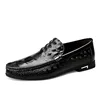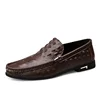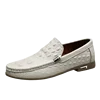# Men Dress Crocodile Pattern High Quality Formal Shoes

৳ 3,677.10৳ 5,953.40 (-38%)

16723 in stock

 colorShoe Size 383940414243444546Clear
SizeHeel to ToeUS SizeChinese Size
36235.536
3723.5637
38246.538
3924.5739
40257.540
4125.5841
4226942
4326.59.543
44271044
4527.510.545
46281146
4728.51247
48291348
4929.51449
50301550
SKU: 4000830328420 Categories: , , ,

Tips:
1. This is NOT Standard EUR US Size.
2. The foot length is the only standard for customers to choose the right size.
3. Insole length should be 0-1.5cm bigger than your foot length.
4. If you are not sure of the correct size, welcome to contact us.

If your foot length is 23cm. Choose size 36 =5.5
If your foot length is 23.5cm. Choose size 37 =6
If your foot length is 24cm. Choose size 38 =6.5
If your foot length is 24.5cm. Choose size 39 =7
If your foot length is 25cm. Choose size 40 =7.5
If your foot length is 25.5cm. Choose size 41 =8
If your foot length is 26cm. Choose size 42 =9
If your foot length is 26.5cm. Choose size 43 =9.5
If your foot length is 27cm. Choose size 44 =10
If your foot length is 27.5cm. Choose size 45 =10.5
If your foot length is 28cm. Choose size 46 =11
If your foot length is 28.5cm. Choose size 47=12
If your foot length is 29cm. Choose size 48 =13
If your foot length is 29.5cm. Choose size 49 =14
If your foot length is 30cm. Choose size 50=15